NEET  >  Electronic Configuration & Wave functions

# Electronic Configuration & Wave functions - Notes | Study Chemistry Class 11 - NEET

 Table of contentsWhat are Electron Configurations?Magnetic moment (m):Radial wave function (R)Radial Probability density (R2)Radial probability functions 4πr2R2Angular wave Function QF.1 Crore+ students have signed up on EduRev. Have you?

What are Electron Configurations?

• The distribution of electrons of an atom in its various shell, subshells and orbitals is known as electron configuration.
• Electron configurations of atoms follow a standard notation in which all electron-containing atomic subshells (with the number of electrons they hold written in superscript) are placed in a sequence.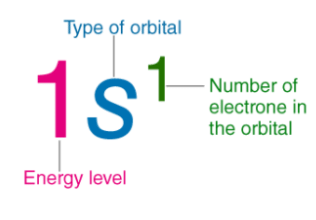• For example, the electron configuration of sodium is 1s22s22p63s1.
• Valence shell or outermost shell → nth shell
• Penultimate shell-Inner to outermost shell → (n -1) shell
• Anti Penultimate shell → (n - 2) shell

Electronic configuration can be expressed in the following ways :

(i) Orbital notation method : nlx

Li → 3 → 1s2 2s1

(ii) Orbital diagram method :

Li → 3 →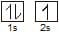(iii) Condensed form :

[He] 2s1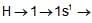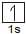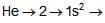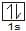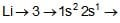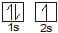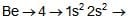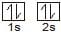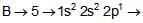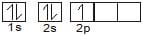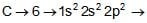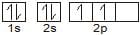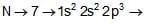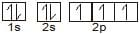Electronic Configuration of ions :

To write the electronic configuration of ions, first, write the electronic configuration of the neutral atom and then add (for a negative charge) or remove (for a positive charge) electrons in the outer shell according to the nature and magnitude of charge present on the ion. e.g.

Al → [Ne]3s2 3p1,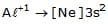,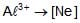Similarly, in the case of transition elements, electrons are removed from nth shell for e.g. 4th shell in case of 3 d series.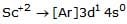Cl : [Ne] 3s2.3p5

Cl- : [Ne] 3s2.3p6

Exceptional configuration:

Expected configuration Cr - 24 -> [Ar] 4s2 3d4

Observed configuration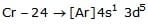Expected configuration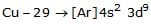Observed configuration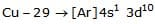Above exceptional configuration can be explained on the basis of following factors.
(i) Symmetrical Electronic Configuration :

It is well-known fact that symmetry leads to stability, orbitals of a subshell having half-filled or full-filled configuration (i.e. symmetrical distribution of electrons) are relatively more stable. This effect is dominating in d and f subshell.

d5, d10, f7, f14 configurations are relatively more stable.

(ii) Exchange Energy :

It is assumed that electrons in degenerate orbitals, do not remain confined to a particular orbital rather it keeps on exchanging its position with electron having same spin and same energy (electrons present in orbitals having same energy). Energy is released in this process known as exchange energy which imparts stability to the atom. More the number of exchanges, more will be the energy released, more the energy released stability will be more.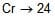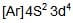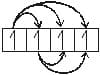→ 3 2 1 = 6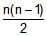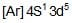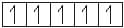where n represents no. of electron having same spin.

The relative value of exchange energy and transfer energy (4s →3d) decides the final configuration. For example, if magnitude of Dep. energy Ex. energy > transfer energy as in cases of Cu and Cr then configuration is: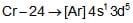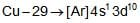respectively.

In case of carbon magnitude of transfer energy > dep. energy exch. energy and configuration of carbon is: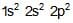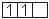Magnetic moment (m):

It is the measure of the magnetic nature of substance

where n is no. of unpaired electrons.

When m = 0, then there is no unpaired electron in the species and it is known as diamagnetic species.

These species are repelled by magnetic field.

When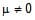, for paramagnetic species. These species have unpaired electrons and are weakly attracted by the magnetic field.

Ques. Calculate magnetic moment of these species

(i) Cr

(i) Cr : No. of unpaired electrons = 6.

=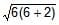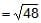BM.

Generally, species having unpaired electrons are coloured or impart colour to the flame. Species having unpaired electrons can be easily excited by the wavelengths corresponding to visible lights and hence they emit radiations having characteristic colour.

In all cases R approaches zero as r approaches infinity. One finds that there is a node in the 2s radial function. In general, it has been found that ns-orbitals have (n-1) nodes, np-orbitals have (n-2) nodes etc.

The importance of these plots lies in the fact that they give information about how the radial wave function changes with distance r and about the presence of nodes where the change of sign of R occurs.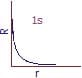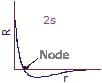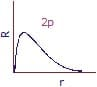Fig: Variation of R with r.

The square of the radial wave function R2 for an orbital gives the radial density. The radial density gives the probability density of finding the electron at a point along a particular radius line. To get such a variation, the simplest procedure is to plot R2 against r. These plots give useful information about probability density or relative electron density at a point as a function of radius. It may be noted that while for s-orbitals the maximum electron density is at the nucleus. all other orbitals have zero electron density at the nucleus.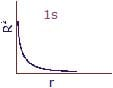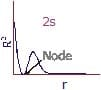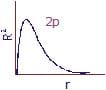Fig: Plot of Rwith r.

The radial density R2 for an orbital, as discussed above, gives the probability density of finding the electron at a point at a distance r from the nucleus. Since the atoms have spherical symmetry, it is more useful to discuss the probability of finding the electron in a spherical shell between the spheres of radius (r dr) and r. The volume of the shell is equal to 4/3π(r dr)3-4/3πr3 = 4πr2dr.

This probability which is independent of direction is called radial probability and is equal to 4πr2drR2.

Radial probability function (4πr2drR2) gives the probability of finding the electron at a distance r from the nucleus regardless of direction.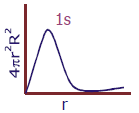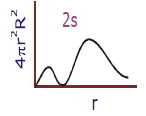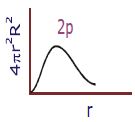The radial probability distribution curves obtained by plotting radial probability functions versus distance r from the nucleus for 1s, 2s and 2p orbitals are shown in fig.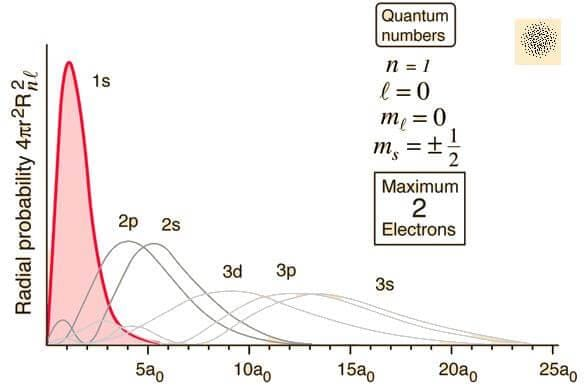Hydrogen 3s Radial Probability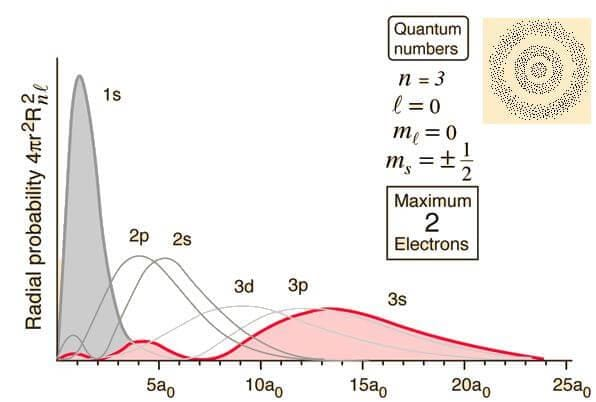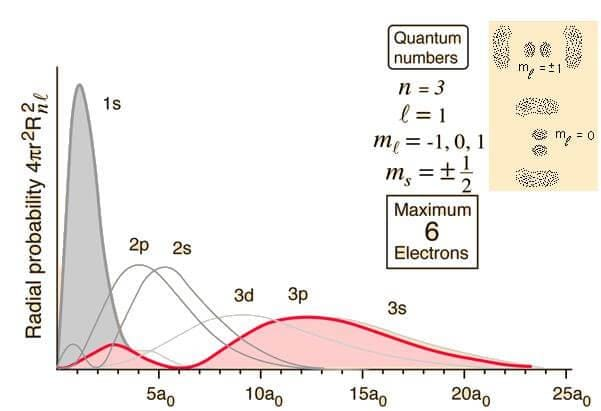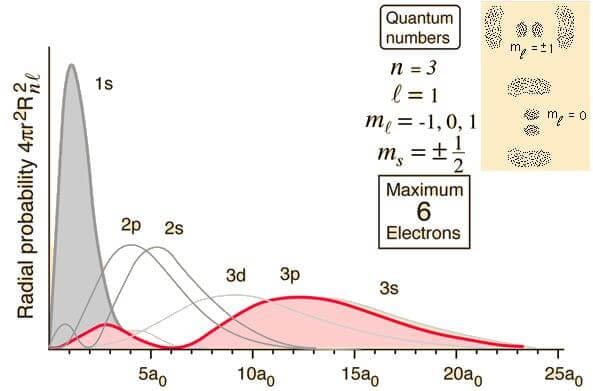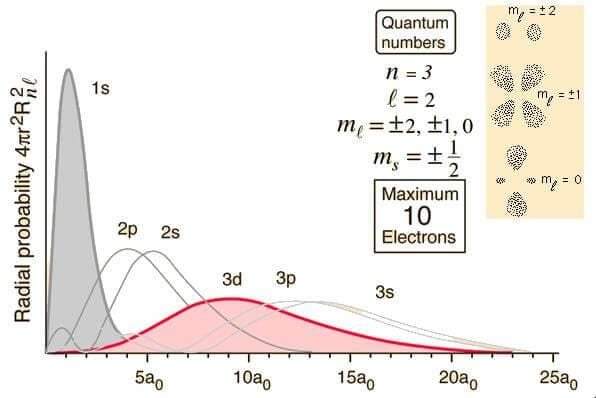Angular wave Function QF.

The angular wave function ' Q F ' depends only on the quantum number l and ml and is independent of the principal quantum number n for a given type of orbital. It therefore means that all s orbitals will have same angular wave function.

(A) Angular Wave Function, ' Q F '

For an s-orbital, the angular part is independent or angle and is, therefore, of constant value. Hence this graph is circular or, more properly, in three dimensions i.e., spherical. For the pz orbital, we get two tangent spheres. The px and py orbitals are identical in shape but are oriented along the x and y axes respectively. The angular, wave function plots for d and f-orbitals are four lobed and six-lobed respectively.

It is necessary to keep in mind that in the angular wave function plots, the distance from the center is proportional to the numerical values of ' Q F ' in that direction and is not the distance from the center of the nucleus.

(B) Angular Wave Function, | Q F |2

The angular probability density plots can be obtained by squaring the angular function plots shown in fig. On squaring, different orbitals change in different ways. For an s orbital, the squaring causes no change in shape since the function everywhere is the same: thus another sphere is obtained.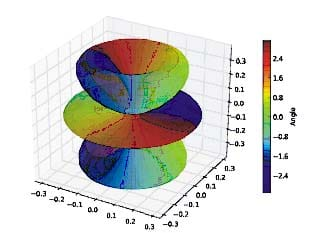Fig: Angular wave function.

The document Electronic Configuration & Wave functions - Notes | Study Chemistry Class 11 - NEET is a part of the NEET Course Chemistry Class 11.
All you need of NEET at this link: NEET

## Chemistry Class 11

204 videos|331 docs|229 tests

## Chemistry Class 11

204 videos|331 docs|229 tests

### How to Prepare for NEET

Read our guide to prepare for NEET which is created by Toppers & the best Teachers

Track your progress, build streaks, highlight & save important lessons and more!

,

,

,

,

,

,

,

,

,

,

,

,

,

,

,

,

,

,

,

,

,

;